# Cramer’s Rule

## What is Cramer’s Rule and how to solve linear equation problems using Cramer’s rule?

In mathematics, to solve linear equations, we use matrix form. Matrix is the rectangular array of elements enclosed in brackets “[]”. There are some methods and rules of matrix used to solve the linear equations. Creamer’s rule is one of them.

Determinants are also used in this rule. In this post, we will learn the basic concept of this rule and use some examples to understand how to solve the problems of linear equations by using Cramer’s rule.

## What is Cramer’s Rule?

In matrices, Cramer’s rule is used to find the values of the unknown variables present in the linear equations. Cramer’s rule is used to find the system of the equations and the equations must be linear.

In this method, all the calculations are based on determinants so this method is also named the determinant method. The rectangular array of matrices is not used in Cramer’s rule, so we use the square matrix form. In the square matrix, there is an equal number of rows and columns are present.

Since the definition of the matrix states that the rectangular array of the matrix then the question must arise in the mind why do we use the square matrix for Cramer’s rule? The answer is that all the square matrices are rectangular matrices in general. So, we always conclude that the square matrix is rectangular.

## The formula of Cramer’s rule

Cramer’s rule is following the equation such,

Ax = b

In this equation, A is the square matrix having an equal number of rows and columns, b is the right-hand side of the linear equation, and X is the unknown variables to be found.

The equations are taken in general in the form of.

A1x + B1y = C1

A2x + B2y = C2

The matrix form of this general linear equation, along with x-matrix and y-matrix is given by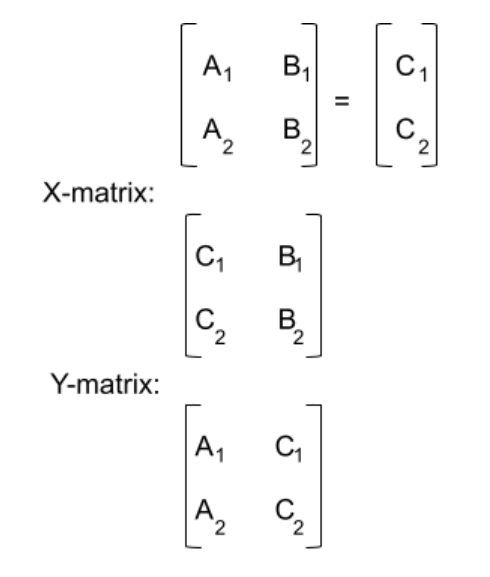The first step of Cramer’s rule is to convert the matrix into matrix form. And then find the determinant of all the square matrices that we made. In Cramer’s rule, if the determinant is zero of a square matrix, then this rule is not applicable.

The following forms are used to calculate the unknown variables x and y of the linear equations.

To determine the value of x

X = det[x]/det

To determine the value of y

y = det[y]/det

## How to solve linear equation problems by using Cramer’s Rule?

To solve the linear equation problems, you must be familiar with the basics formula and working of Cramer’s rule. In Cramer’s rule, determinants are used, determinants are to be calculated by applying “||” for matrices instead of [] brackets.

There is an online tool Cramer’s rule calculator used for such calculations to reduce the difficulty of large calculations.

The steps to determine the determinant for 2x2 matrices are very simple just follow the given method.

abcd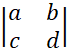= (a x d) – (b x c)

The steps to determine the determinant for 3x3 matrices are pretty difficult and lengthy just follow the given method.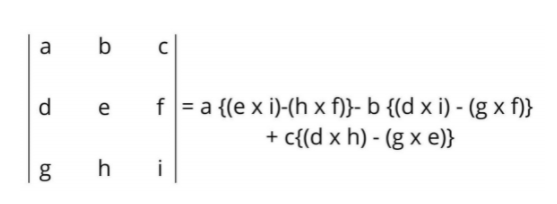### Example 1

Find the unknowns of the linear equation by using Cramer’s rule.

5x + 3y = 6

x + 3y = 2

Solution

Step 1: First write in AX = B form.

5313x y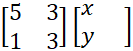= 6 2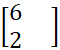Step 2: Find the determinant of square matrix A.

A = 5313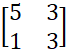Step 3: Write the formula to determine the determinant of square matrix A.

abcd= (a x d) – (b x c)

Step 4: Place the values of each term in the above formula.

5313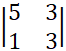= (5 x 3) – (3 x 1)

5313= 15 – 3

5313= 12

Det A = 12

Step 5: Now take the values of b in the first column of matrix A and give the name of x value.

X values = 6323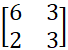Step 6: Find the determinant of the above matrix.

6323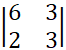= (6 x 3) – (3 x 2)

6323= 18 – 6

6323= 12

detx = 12

Step 7: Now take the values of b in the second column of matrix A and give the name of y value.

X values = 5612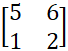Step 8: Find the determinant of the above matrix.

5612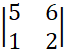= (5 x 2) – (6 x 1)

5612= 10 – 6

5612= 4

dety = 4

Step 9: By the formula of Cramer’s rule to find the unknown variables, we have.

X = detx/det

Y = dety/det

Step 10: Place the values of the calculated determinants in the above formulas.

X = 12/12 = 1

Y = 4/12 = 1/3 = 0.3333

### Example 2

Find the unknowns of the linear equation by using Cramer’s rule.

5x – 4y = 6

3x + 9y = 2

Solution

Step 1: First write in AX = B form.

5-439x y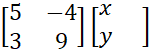= 6 2Step 2: Find the determinant of square matrix A.

A = 5-439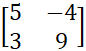Step 3: Write the formula to determine the determinant of square matrix A.

abcd= (a x d) – (b x c)

Step 4: Place the values of each term in the above formula.

5-439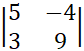= (5 x 9) – (3 x (-4))

5-439= 45 – (-12)

5-439= 45 + 12

5-439= 57

Det A = 57

Step 5: Now take the values of b in the first column of matrix A and give the name of x value.

X values = 6-429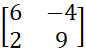Step 6: Find the determinant of the above matrix.

6-429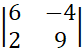= (6 x 9) – (-4 x 2)

6-429= 54 – (-8)

6-429= 54 + 8

6-429= 62

detx = 62

Step 7: Now take the values of b in the second column of matrix A and give the name of y value.

X values = 5632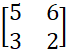Step 8: Find the determinant of the above matrix.

5632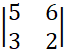= (5 x 2) – (6 x 3)

5632= 10 – 18

5632= -8

dety = -8

Step 9: By the formula of Cramer’s rule to find the unknown variables, we have.

X = detx/det

Y = dety/det

Step 10: Place the values of the calculated determinants in the above formulas.

X = 62/57 = 1.0877

Y = -8/57 = -0.1404

## Summary

Now you grab all the basis of Cramer’s rule. The working of this rule is very simple, just some practice is required, this rule is also defined in the vedic maths. From these given examples, you can also solve Cramer’s rule for 3x3 matrices by following the same steps.